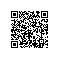# 经典算法题每日演练——第三题 猴子吃桃

猴子第一天摘下若干个桃子，当即吃了一半，还不过瘾就多吃了一个。第二天早上又将剩下的桃子吃了一半，还是不过瘾又多

我们找到递推公式，问题就迎刃而解了。

令S10=1，容易看出 S9=2(S10+1)， 简化一下

S9=2S10+2

S8=2S9+2

.....

Sn=2Sn+1+2

class Program
{
static void Main(string[] args)
{
int sum = SumPeach(1);

Console.WriteLine("第一天摘得桃子有:{0}", sum);

}

//递归
static int SumPeach(int day)
{
if (day == 10)
return 1;

return 2 * SumPeach(day + 1) + 2;
}
}

class Program
{
static void Main(string[] args)
{
int sum = SumPeachTail(1, 1);

Console.WriteLine("第一天摘得桃子有:{0}", sum);

}

//尾递归
static int SumPeachTail(int day, int total)
{
if (day == 10)

//将当前的值计算出传递给下一层
return SumPeachTail(day + 1, 2 * total + 2);
}
}1.  代换法。

2.  递归树法。

3.  主定理。

①：猜一下递归式复杂度的上界或者下界。

②：用数学归纳法证明你的复杂度是正确的。

Tn=2Tn-1+2             ①

Tn<=cO(n)=cn           ③

③代入①知

Tn<=2c(n-1)+2=2cn-2c+2

=cn-c+1

=cn-(c-1)使用钉钉扫一扫加入圈子
+ 订阅